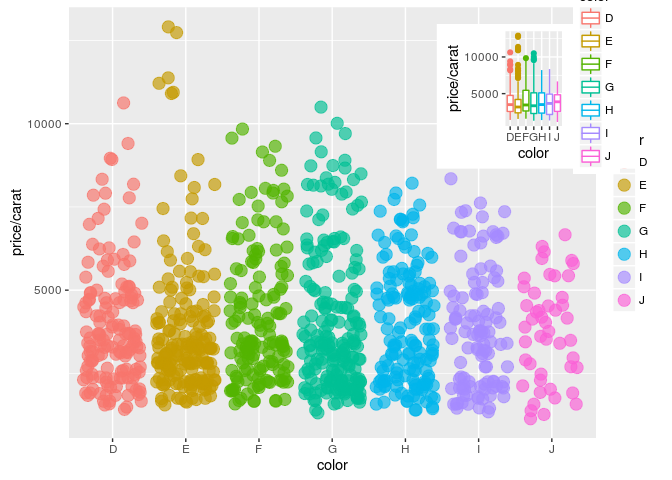• What is `ggplot2`?
• High-level graphics system
• Implements grammar of graphics from Leland Wilkinson
• Streamlines many graphics workflows for complex plots
• Syntax centered around main `ggplot` function
• Simpler `qplot` function provides many shortcuts
• Documentation and Help

## `ggplot2` Usage

• `ggplot` function accepts two arguments
• Data set to be plotted
• Aesthetic mappings provided by `aes` function
• Additional parameters such as geometric objects (e.g. points, lines, bars) are passed on by appending them with `+` as separator.
• List of available `geom_*` functions see here
• Settings of plotting theme can be accessed with the command `theme_get()` and its settings can be changed with `theme()`.
• Preferred input data object
• `qplot`: `data.frame` (support for `vector`, `matrix`, `...`)
• `ggplot`: `data.frame`
• Packages with convenience utilities to create expected inputs
• `plyr`
• `reshape`

## `qplot` Function

The syntax of `qplot` is similar as R’s basic `plot` function

• Arguments
• `x`: x-coordinates (e.g. `col1`)
• `y`: y-coordinates (e.g. `col2`)
• `data`: data frame with corresponding column names
• `xlim, ylim`: e.g. `xlim=c(0,10)`
• `log`: e.g. `log="x"` or `log="xy"`
• `main`: main title; see `?plotmath` for mathematical formula
• `xlab, ylab`: labels for the x- and y-axes
• `color`, `shape`, `size`
• `...`: many arguments accepted by `plot` function

## `qplot`: scatter plot basics

Create sample data

Simple scatter plot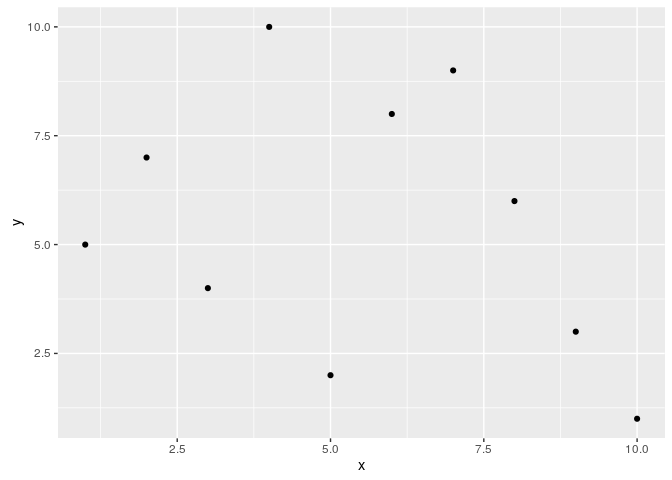Prints dots with different sizes and colors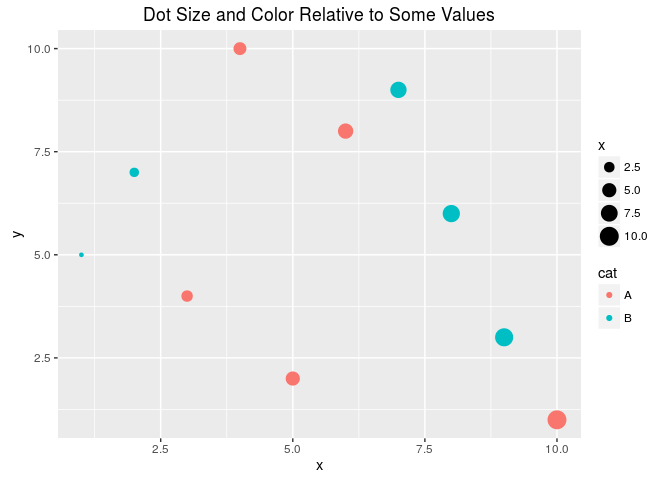Drops legend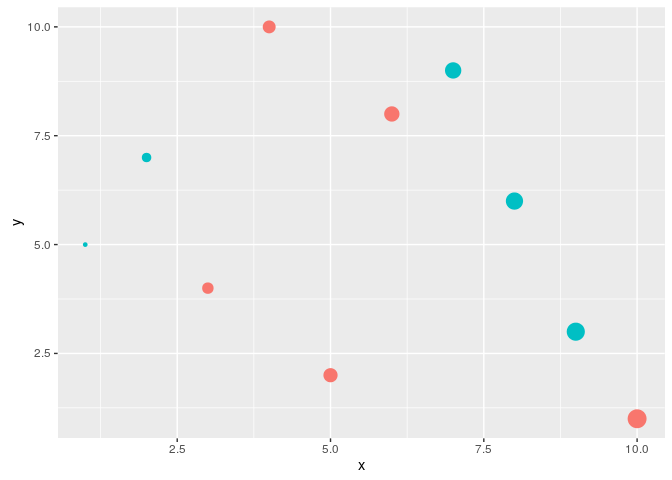Plot different shapes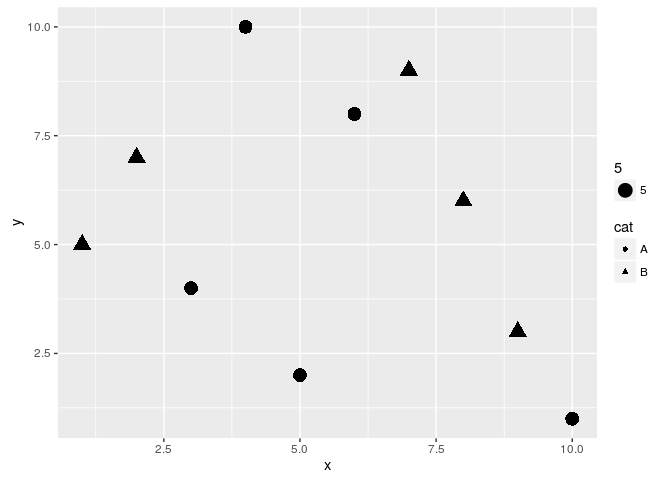### Colored groups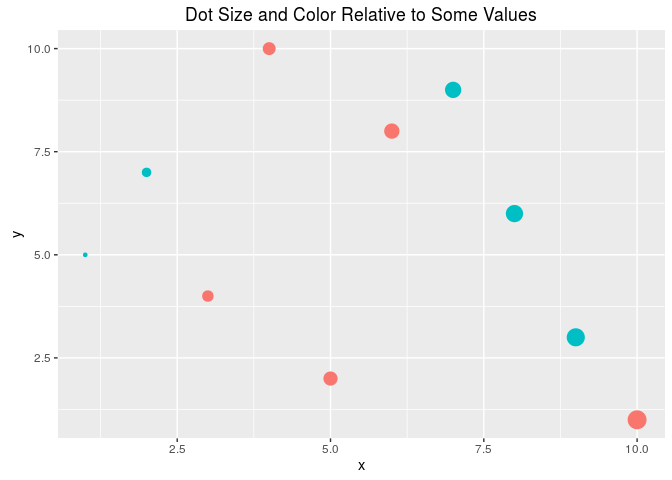### Regression line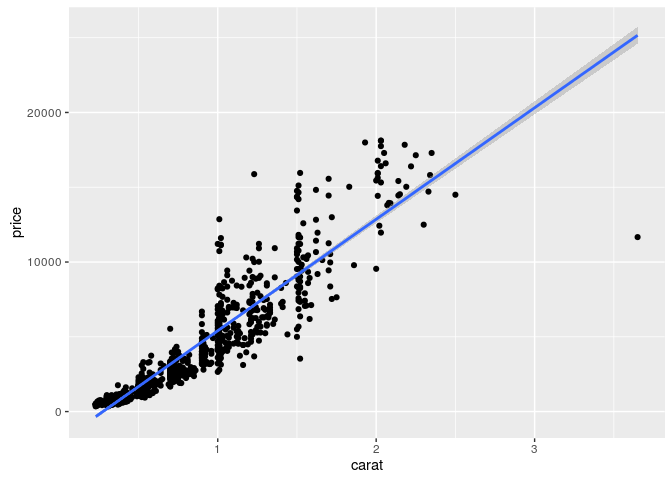### Local regression curve (loess)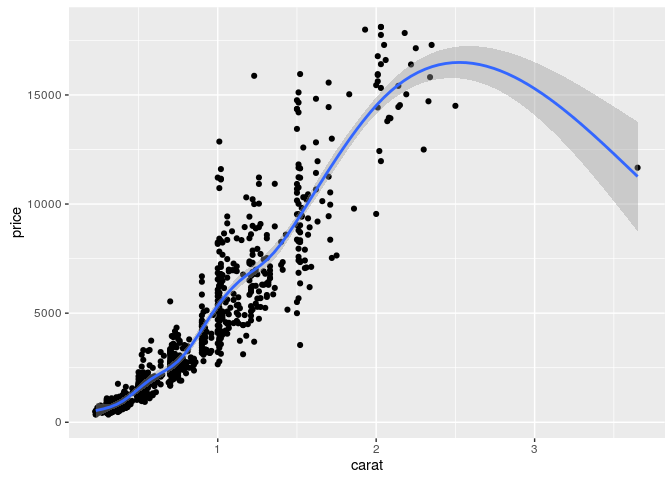## `ggplot` Function

• More important than `qplot` to access full functionality of `ggplot2`
• Main arguments
• data set, usually a `data.frame`
• aesthetic mappings provided by `aes` function
• General `ggplot` syntax
• `ggplot(data, aes(...)) + geom() + ... + stat() + ...`
• Layer specifications
• `geom(mapping, data, ..., geom, position)`
• `stat(mapping, data, ..., stat, position)`
• `scales`
• `coordinates`
• `facet`
• `aes()` mappings can be passed on to all components (`ggplot, geom`, etc.). Effects are global when passed on to `ggplot()` and local for other components.
• `x, y`
• `color`: grouping vector (factor)
• `group`: grouping vector (factor)

### Changing Plotting Themes in `ggplot`

• Theme settings can be accessed with `theme_get()`
• Their settings can be changed with `theme()`

Example how to change background color to white

### Storing `ggplot` Specifications

Plots and layers can be stored in variables

Returns information about data and aesthetic mappings followed by each layer

Print dots with different sizes and colors

Syntax to pass on other data sets

Saves plot stored in variable `p` to file

## `ggplot`: scatter plots

### Basic example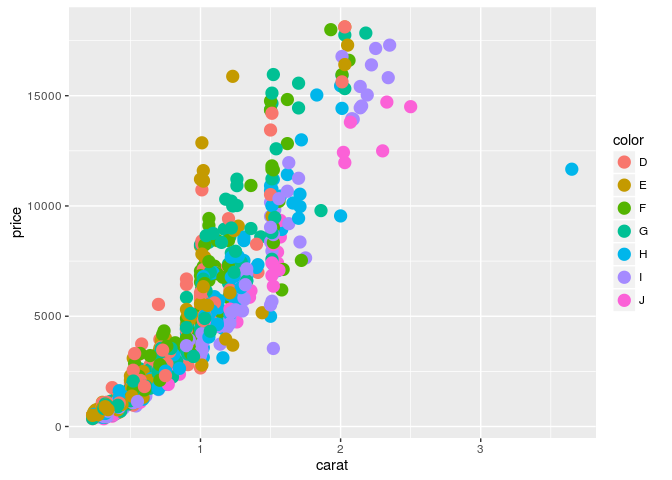### Regression line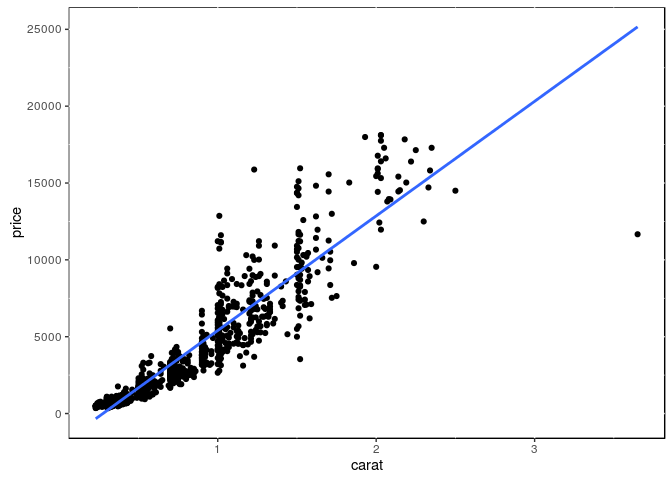### Several regression lines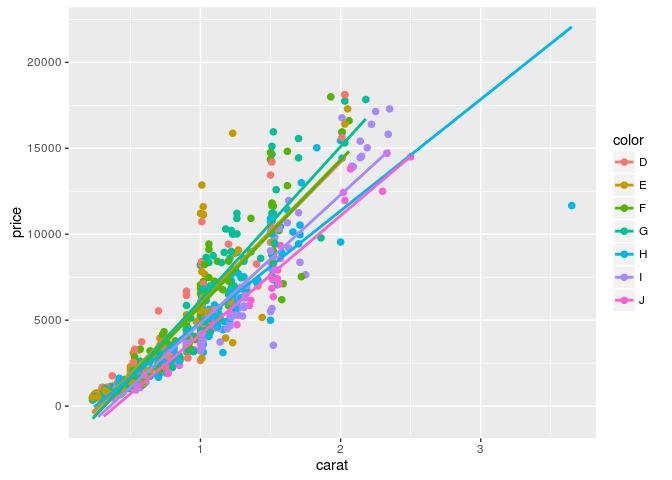### Local regression curve (loess)## `ggplot`: line plot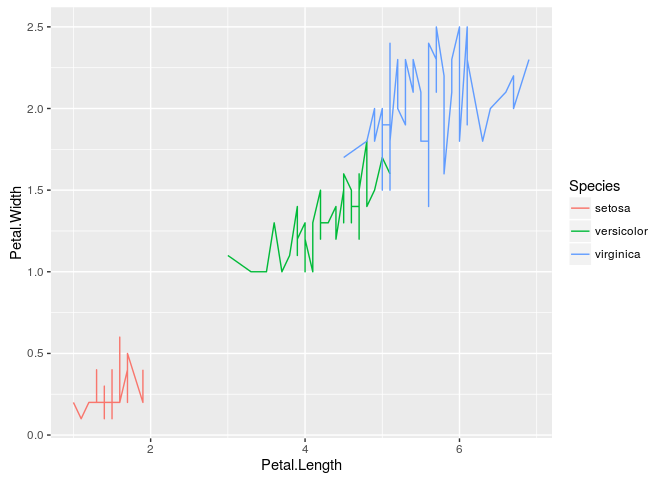## Faceting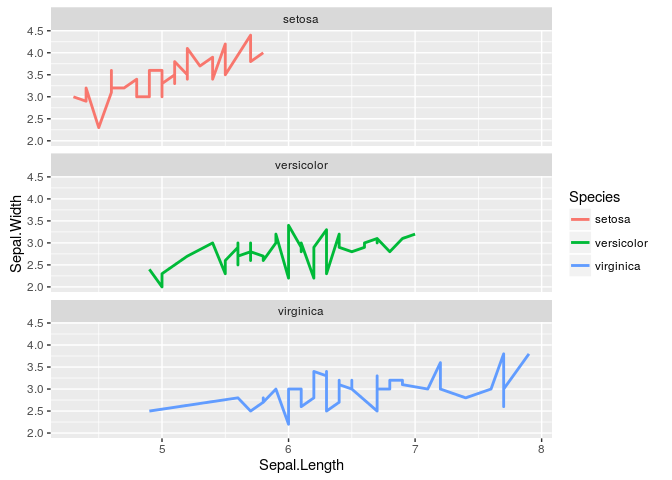### Exercise 3

Scatter plots with `ggplot2`

• Task 1: Generate scatter plot for first two columns in \Rfunction{iris} data frame and color dots by its \Rfunction{Species} column.
• Task 2: Use the \Rfunarg{xlim, ylim} functionss to set limits on the x- and y-axes so that all data points are restricted to the left bottom quadrant of the plot.
• Task 3: Generate corresponding line plot with faceting show individual data sets in saparate plots.

Structure of `iris` data set

## Bar plots

Sample Set: the following transforms the `iris` data set into a ggplot2-friendly format.

Calculate mean values for aggregates given by `Species` column in `iris` data set

Calculate standard deviations for aggregates given by `Species` column in `iris` data set

Reformat `iris_mean` with `melt`

Reformat `iris_sd` with `melt`

Define standard deviation limits

### Verical orientation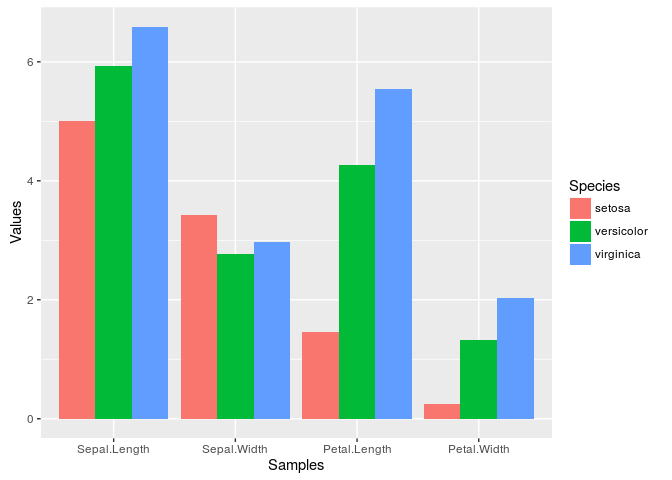### Horizontal orientation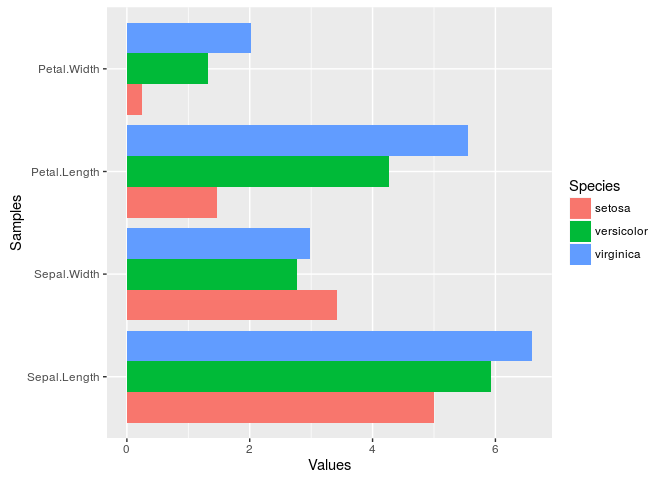### Faceting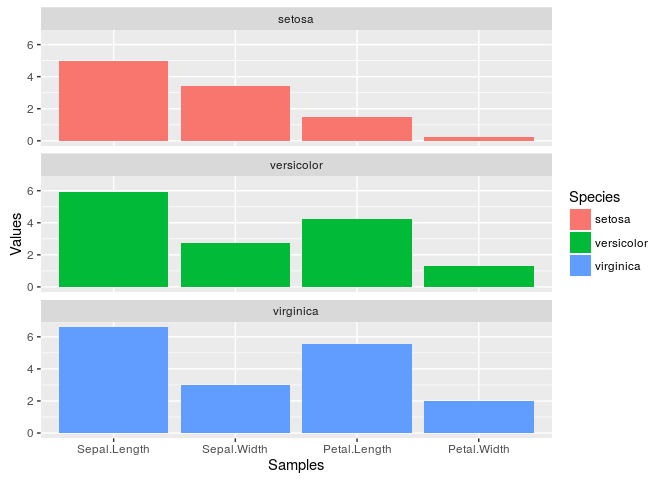### Error bars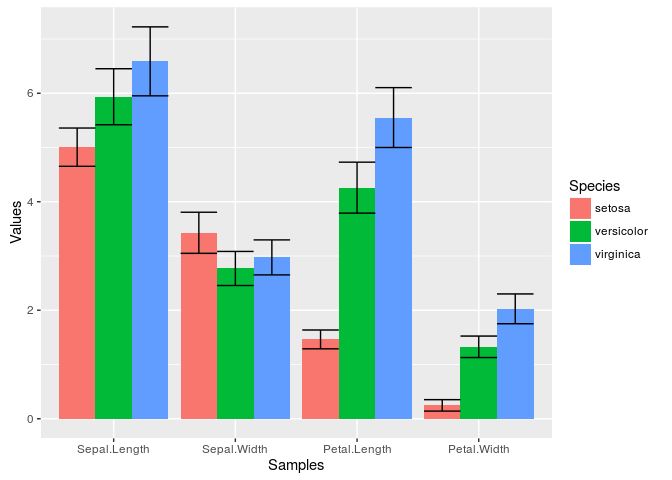### Mirrored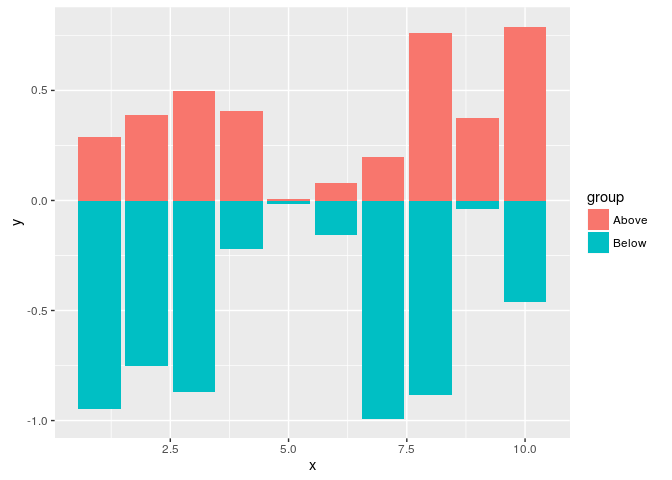## Changing Color Settings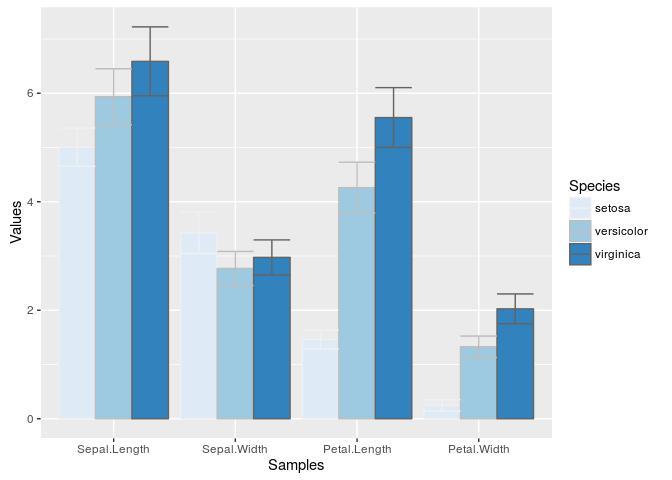### Using standard colors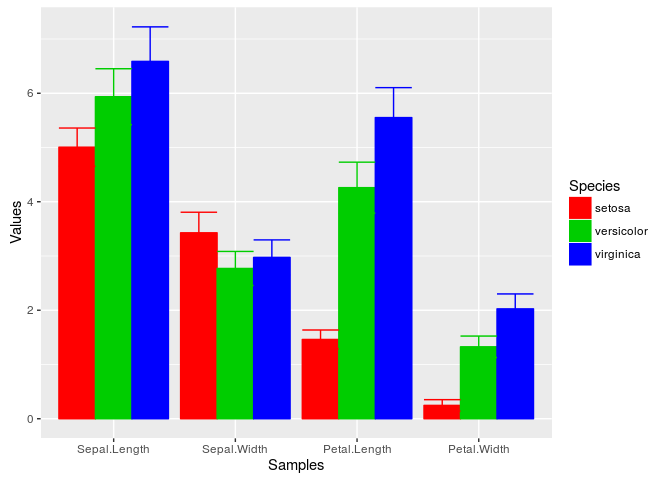### Exercise 4

Bar plots

• Task 1: Calculate the mean values for the `Species` components of the first four columns in the `iris` data set. Use the `melt` function from the `reshape2` package to bring the data into the expected format for `ggplot`.
• Task 2: Generate two bar plots: one with stacked bars and one with horizontally arranged bars.

Structure of iris data set

## Data reformatting example

Here for line plot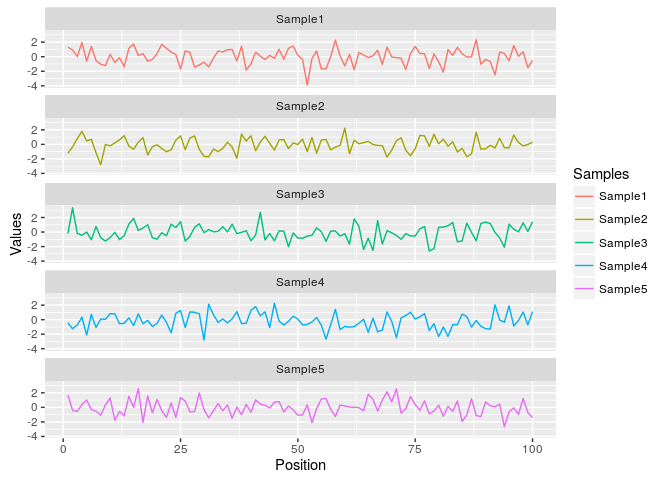Same data can be represented in box plot as follows

## Jitter Plots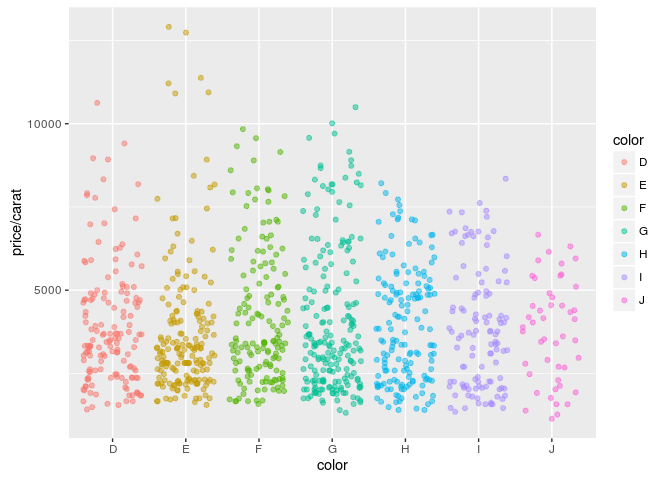## Box plots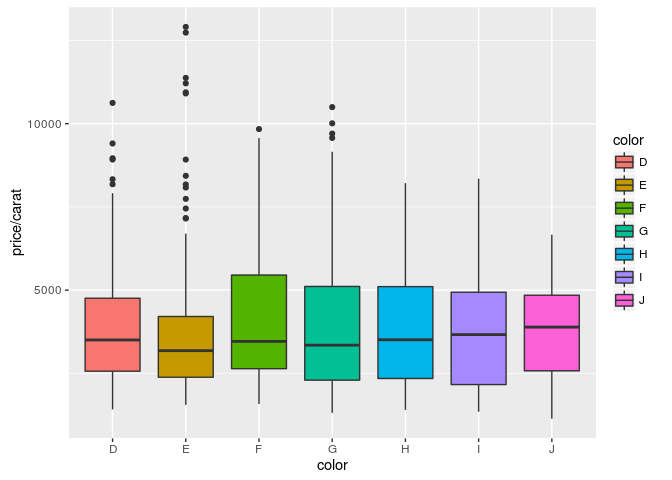## Density plots

### Line coloring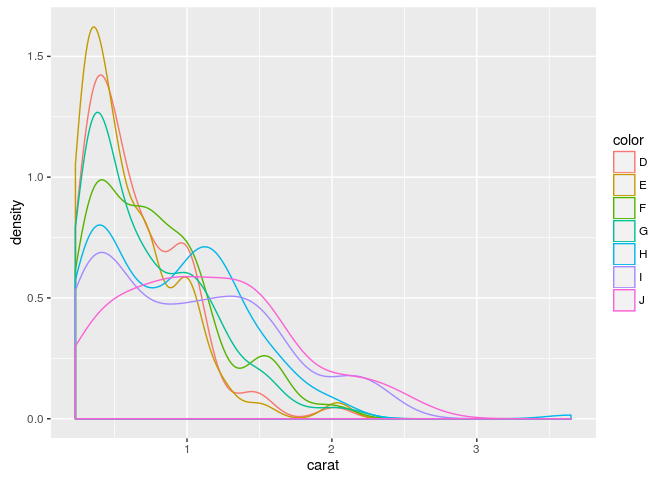### Area coloring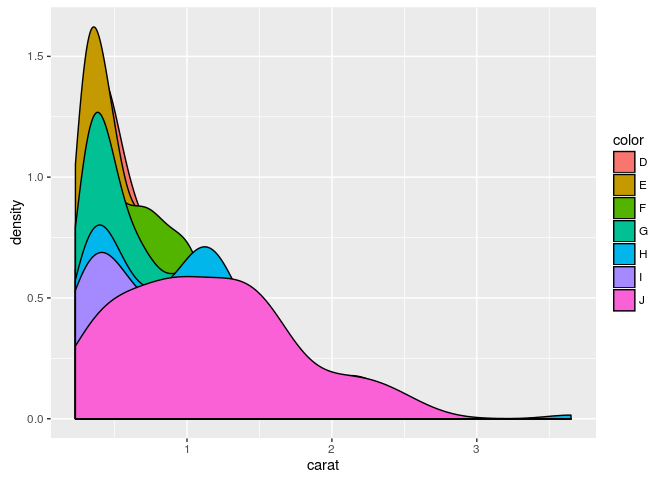## Histograms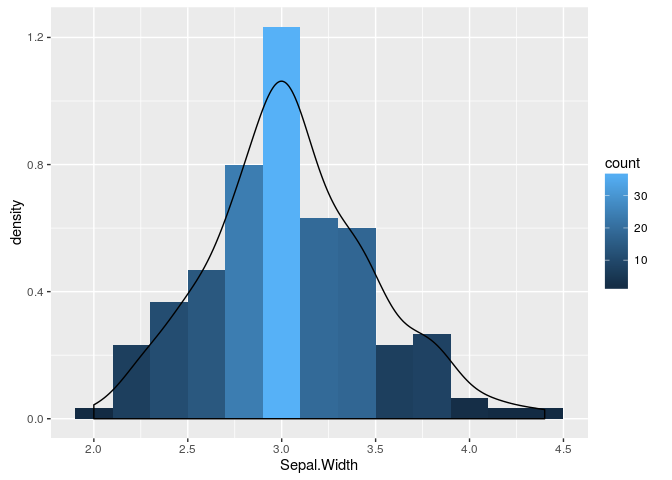## Pie Chart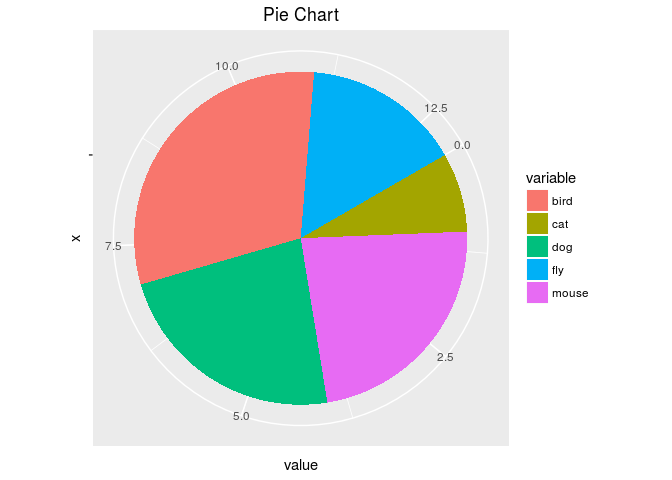## Wind Rose Pie Chart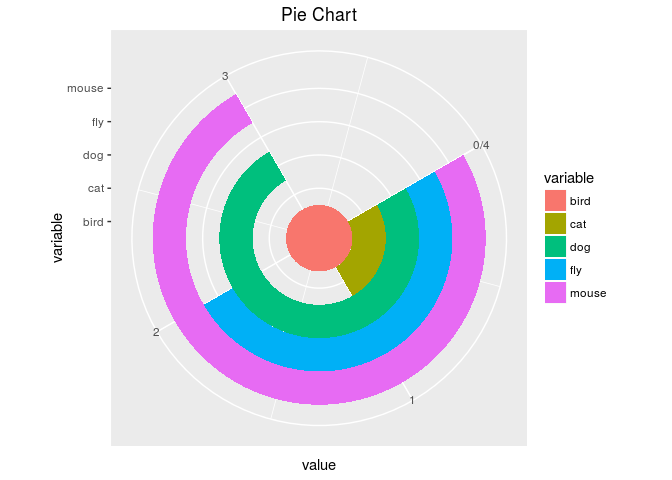## Arranging Graphics on Page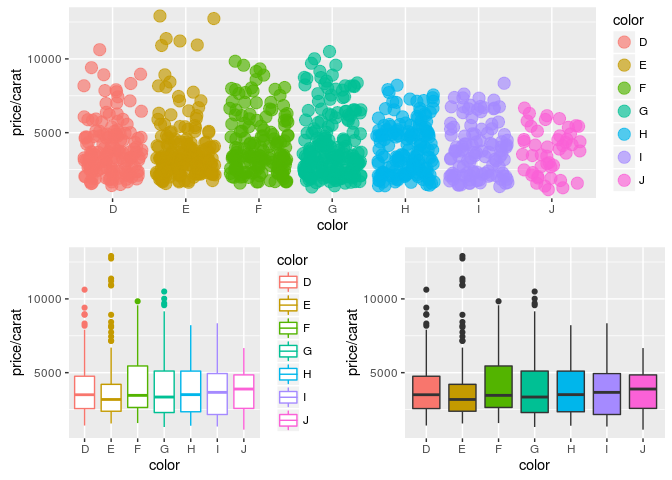## Inserting Graphics into Plots